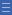## Featured resource

Home > Topdrawer > Mental computation > Activities > Renaming to add and subtract

# Renaming to add and subtract

Combining 10 ones to form a new 10 or decomposing a 10 into ones are procedures. Automation of these renaming procedures eases the memory load in more complex calculations.

Pose addition and subtraction problems -- in context -- that can be solved using place value with renaming.

In addition problems this involves combining ones to form a ten.

For example, 57 + 28 = ? requires combining 7 and 8 to form 10 and 5.

You can watch the Renaming Tens with Addition video.

In subtraction we decompose tens when more ones need to be taken away than are immediately available.

For example, 83 – 47 = ? requires subtraction of seven ones when only three are immediately available.

You can watch the Renaming Tens with Subtraction video.

Both of these videos illustrate renaming strategies based on splitting tens. Renaming problems can also be solved using jumping strategies. Jumping tends to be more reliable as a mental strategy because it removes the memory load associated with the starting number.

Jumping strategies can be practised using the empty number line applet on the New Zealand Maths website.

These ideas are explored further in Number Facts: Addition and Subtraction and Fluency and Flexibility.

Yes

Yes

Name Class SectionYear 3: Apply place value to partition, rearrange and regroup numbers to at least 10 000 to assist calculations and solve problems Infobox 3Year 2: Solve simple addition and subtraction problems using a range of efficient mental and written strategies Infobox 3Source Infobox 3